## Accelerated Prompt Gamma estimation for clinical Proton Therapy simulations

November 11, 2016

Abstract

Purpose: There is interest in the particle therapy community to use prompt gammas (PG), a natural byproduct of particle treatment, for range veriﬁcation and eventually dose control. However, PG production is a rare process and therefore estimating PGs exiting a patient during a proton treatment plan executed by a Monte Carlo (MC) simulation converges slowly. Recently, diﬀerent approaches to accelerating the estimation of PG yield have been presented. Sterpin et al. (2015) described a fast analytic method which is still sensitive to heterogeneities. El Kanawati et al. (2015) described a variance reduction method (pgTLE) that accelerates the PG estimation by precomputing PG production probabilities as a function of energy and target materials, but has as drawback that the proposed method is limited to analytical phantoms.

Materials and Methods: We present a two-stage variance reduction method, named voxelized pgTLE (vpgTLE) that extends pgTLE to voxelized volumes. As a preliminary step, PG production probabilities are precomputed once and stored in a database. In stage one, we simulate the interactions between the treatment plan and the patient CT with low statistic MC to obtain the spatial and spectral distribution of the PGs. As primary particles are propagated throughout the patient CT, the PG yields are computed in each voxel from the initial database, as function of the current energy of the primary, the material in the voxel and the step length. The result is a voxelized image of PG yield, normalized to a single primary. The second stage uses this intermediate PG image as a source to generate and propagate the number of PGs throughout the rest of the scene geometry, e.g. into a detection device, corresponding to the number of primaries desired.

Results: We achieved a gain of around $1{0}^{3}$ for both a geometrical heterogeneous phantom and a complete patient CT treatment plan with respect to analog MC, at a convergence level of 2% relative uncertainty in the 90% yield region. The method agrees with reference analog MC simulations to within $1{0}^{-4}$, with negligible bias. Gains per voxel range from $1{0}^{2}$ to $1{0}^{4}$.

Conclusion: The presented generic PG yield estimator is drop-in usable with any geometry and beam conﬁguration. We showed a gain of three orders of magnitude compared to analog MC. With a large number of voxels and materials, memory consumption may be a concern and we discuss the consequences and possible trade-oﬀs. The method is available as part of Gate 7.2.

### 1 Introduction

The well-deﬁned range of particles in matter is the main reason they are used in cancer treatment today. Unfortunately we are not able to take full advantage of this property, because of uncertainties in patient positioning, uncertainties on the proton range due to unknown displacements or deformations of organs, ill-deﬁned lung, bowel or bladder ﬁlling, and the inherent uncertainty in the Houndsﬁeld unit to particle stopping power conversion. Often, medical practice is to plan conservatively, namely adding margins around the tumor, greatly reducing the potential beneﬁts of particle treatment (Knopf and Lomax2013). Some form of in-vivo, online monitoring is generally considered to be a way out of this predicament. Online monitoring would make measurements of uncertainties such as mentioned above possible, and thereby permit more precise planning which could take maximum advantage of the steep Bragg Peak (BP) fall-oﬀ and reduce damage to tissues surrounding the tumor.

One way to perform monitoring is to use prompt gammas (PGs), a natural byproduct in particle treatments. PGs oﬀer the potential of monitoring treatment at the spot level (Roellinghoﬀ et al.2014Smeets et al.2012) and are therefore of prime interest (Golnik et al.2014Gueth et al.2013Janssen et al.2014Moteabbed et al.2011). These particles are produced in the inelastic nuclear collisions between the incident particle and the medium (tissue) it is traveling through, and they correlate very well with dose deposition. This has been experimentally demonstrated for protons by Min et al. (2006) and for carbon by Testa et al. (2008). The latter also showed that adding Time of Flight (ToF) measurement enables the discrimination between neutron-induced gammas and PGs, greatly cleaning up the signal, which was conﬁrmed by Biegun et al. (2012); Lopes et al. (2015); Pinto et al. (2015); Roellinghoﬀ et al. (2014); Smeets et al. (2012); Verburg and Seco (2014). The authors conclude that online range monitoring is possible, once a detector with suﬃciently large solid angle can be realized. In Fall 2015, at OncoRay in Dresden, Germany, a knife-edge PG camera (Perali et al.2014Richter et al.2016) was put into clinical operation. In addition to geometrical collimation, work is being done on designs that exploit Compton scattering to record the PG signal (Kurosawa et al.2012Roellinghoﬀ et al.2011)

Another approach is to use the fact that in certain nuclear reactions PGs with speciﬁc energies are produced (Verburg and Seco2014Verburg et al.2013). The composition of the produced PG spectrum is dependent on material and proton energy. Certain PG energies tend to be produced close to the BP; that is to say, the associated cross sections are largest when the primary is near the end of its range. Analysis of these spectral lines at given positions may provide suﬃcient information to deduce both the material composition and BP position. Verburg et al. exploit this fact to reconstruct the spatial location of the BP falloﬀ position with a single collimated detector placed at the end of the primary range. Then there is an attempt (Golnik et al.2014) to use the travel time of the primary to reconstruct and control the PG range. Using a camera with high timing resolution and a short, pulsed beam, the ToF between the proton leaving the beam nozzle and the PG arriving in the camera can be recorded, and by putting the camera close to the nozzle looking back at the patient, we know that a larger ToF corresponds to more distal interactions, and a smaller ToF to proximal interactions.

Imaging paradigms such as PG detection are validated against experiments, and often also with Monte Carlo (MC) simulations (Golnik et al.2014Gueth et al.2013Janssen et al.2014Moteabbed et al.2011Robert et al.2013). Conventional MC methods propagate particles according to a set of physical processes through materials. The tracking of a particle is broken up into steps, where at each point, a weighted list of all possible next steps is built and one option is selected by a random number. For rarely occurring processes, convergence to the model of the truth to within acceptable statistical error can be slow. PG emission in particle therapy, when viewed on a voxel-by-voxel basis, is also a rare and slowly converging process (Gueth et al.2013Pinto et al.2015Sterpin et al.2015). This has important implications: ﬁrstly for detector designers, secondly for those who simulate, and thirdly for those interested in comparing the two online in, say, clinical conditions. In the ﬁrst case, detectors are optimized to minimize signal loss (see ﬁg. 1) and advanced reconstruction can be employed to maximize the use of information in the signal. Gueth et al. (2013) has demonstrated a method that works around the low PG yield by using machine learning to correlate predeﬁned patient translations (setup errors) to PG output signals, which reduces the time to produce an estimated translation based on the detected PGs. Since convergence to the model of the truth requires long simulation runtimes, we may compensate with variance reduction methods or cutoﬀs in the time, space, or spectral domains (e.g. ﬁxed runtime, larger voxel size, larger spectral bins). One such variance reduction method is MC splitting, where the moment a rare process occurs not one, but multiple possible futures for that process are computed. The weighted total of these futures are then stored, and so the convergence accelerated.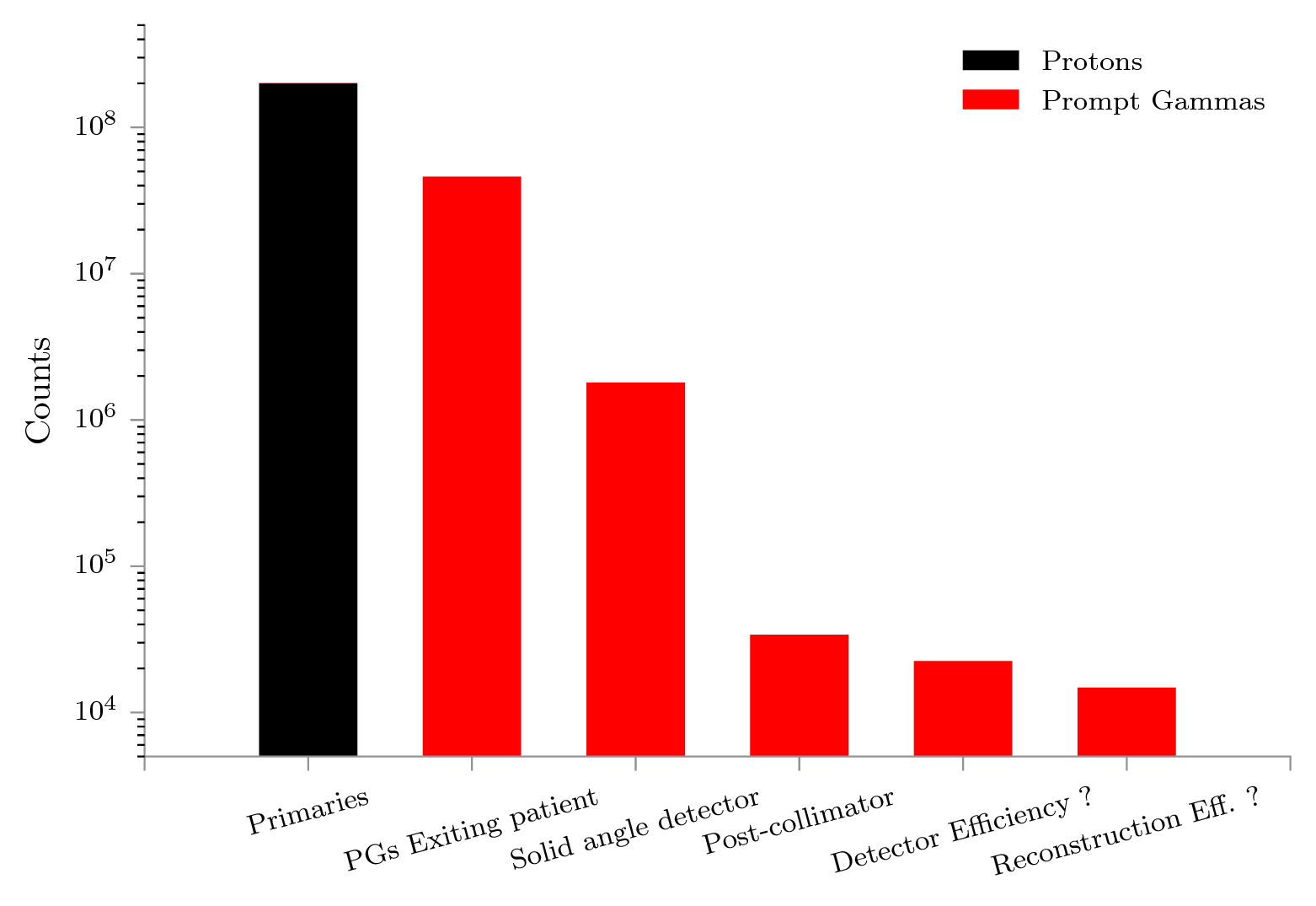Figure 1: Approximate progressive loss of PG signal per spot. The particles were recorded in a simulation with a clinical head and neck CT and associated clinical plan, with a 30-by-30 cm collimator with 43% ﬁll factor, at 40 cm from the patient, with estimated eﬃciencies (66%) for detection and reconstruction. Note this is not the conﬁguration investigated in the rest of this paper.

Recently, a variance reduction method (pgTLE) was described (El Kanawati et al.2015). The PG estimation is accelerated by precomputing PG production probabilities as a function of energy and target materials, with as drawback that it only works for analytical phantoms. Here, we present a two-stage method, voxelized pgTLE (vpgTLE), that extends pgTLE to voxelized volumes, making it useful for clinical simulations. Next we describe the method and give some simulation results in various conﬁgurations. At this time, only protons as primaries have been considered and validated. We ﬁnish with a discussion of background noise estimation, other variance reduction methods and points for improvement.

### 2 Materials and Methods

#### 2.1 A voxelized pgTLE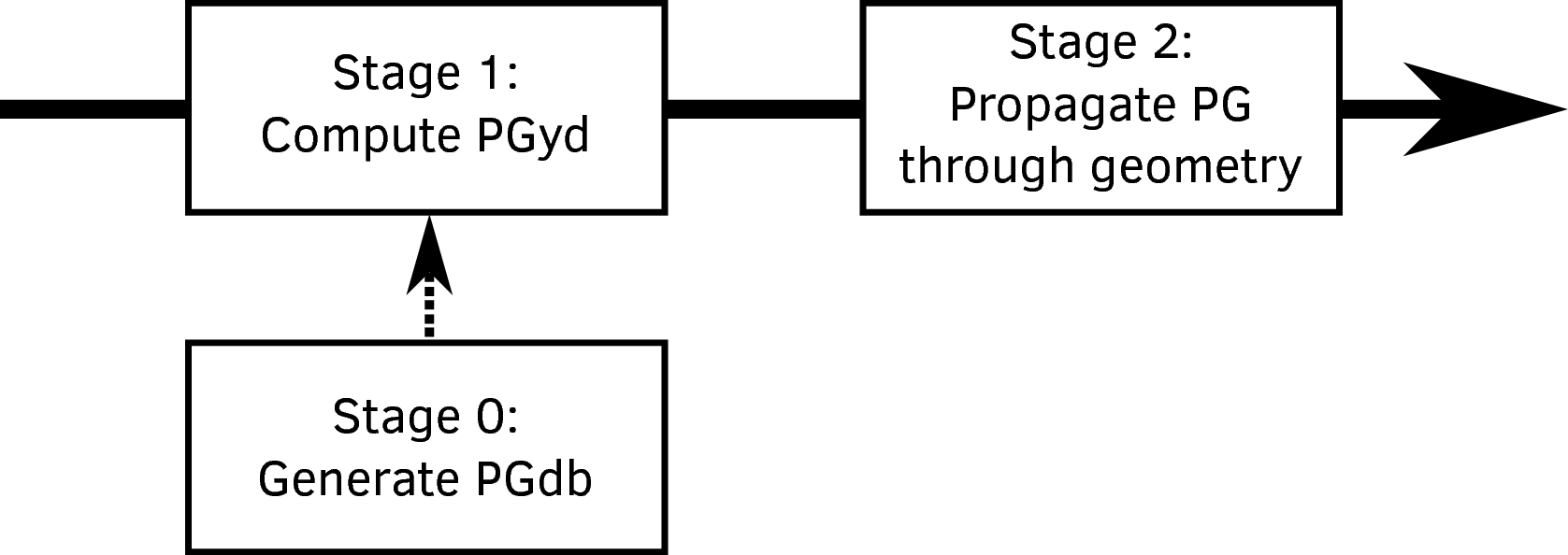Figure 2: Flow chart of the vpgTLE method. Stage 0 is an initial PG database (PGdb) and is computed once. Every subsequent simulation is broken up into Stage 1 and Stage 2. Stage 1 generates a CT speciﬁc PG yield distribution (PGyd) using a limited number of primaries. In Stage 2 the PGyd is used to generate and propagate a representative set of PGs throughout the geometry.

A full voxelized Prompt-Gamma Track Length Estimator (vpgTLE) simulation is broken up into two stages (ﬁg. 2). The process presumes the existence of a prepared database (PGdb) which is an estimate of the eﬀective linear PG production coeﬃcient modulo the density, per element ($\frac{{\Gamma }_{Z}}{{\rho }_{Z}}$, see eq. 1), per PG energy bin per primary energy bin. The PGdb is computed once for a list of common elements, and can then be reused. In stage one, a PG yield distribution image (PGyd) is created, speciﬁc to a particular phantom (or CT-image) and primary source (e.g. a treatment plan, a single spot). This PGyd image stores, per voxel per PG energy bin, the yield per primary. Stage two uses the PGyd and the assumption of isotropic PG emittance to generate and propagate the PGs throughout the rest of the geometry that the user has deﬁned (e.g. the PG detector).

##### 2.1.1 Stage 0: PG Database

First, as explained in El Kanawati et al. (2015), we precalculate a database of PG production probability per PG energy, as a function of element and proton energy. Eq. 1 describes the computed quantity: the PG spectrum $\Gamma$ as a function of proton energy $E$ for a set of elements $Z$. We take the ratio of the number of produced gammas ${N}_{\gamma }$ over the number of inelastic collisions ${N}_{inel}$, scaled by the linear element attenuation coeﬃcient ${\kappa }_{inel}$, related to the proton inelastic nuclear process. We normalize with the density $\rho$ of element $Z$. Bold symbols represent vectors as function of PG energy. We compute the PGdb for protons up to 200MeV in 250 bins.

 $\frac{{\Gamma }_{Z}\left(E\right)}{{\rho }_{Z}}=\frac{{N}_{\gamma }\left(Z,E\right)}{{N}_{inel}\left(Z,E\right)}\frac{{\kappa }_{inel}\left(Z,E\right)}{{\rho }_{Z}}$ (1)

Currently, PG emission models and cross sections implemented in various MC codes (Geant4, Fluka, MCNPX) are still evolving as diﬀerences have been observed between experimental PG data and simulations (Pinto et al.2015), and between MC codes (Pinto et al.2016Robert et al.2013Verburg et al.2012) and research is ongoing to improve accuracy. Hence, if cross sections or implementations of models are updated, the database must be recomputed. In particular, when comparing between simulations, such as in this study between vpgTLE and a reference analog MC, the same physics list must be used.

##### 2.1.2 Stage 1: Compute phantom-speciﬁc PG yield distribution

By performing a low statistic MC, an estimate of the proton track lengths per voxel per proton energy bin is obtained, hence "Track Length Estimation". Note that "track" corresponds to "step" in Geant4 terminology. Together with the eﬀective linear PG production coeﬃcient, the PGyd image is obtained.

Before the simulation starts, we query the CT image for a list of present materials and build ${\Gamma }_{m}$ for each material $m$ encountered. We compute the PGdb in terms of elements, rather than materials, to give the user the option to add new materials or modify the composition of existing ones without recomputing the PGdb. In eq. 2, we sum ${\Gamma }_{Z}$ over all $k$ constituent elements $Z$ in material ${m}_{v}$, weighted by density fraction ${\omega }_{k}$ of element $Z$ in ${m}_{v}$, and scaled by density ${\rho }_{{m}_{v}}$.

 ${\Gamma }_{m}\left(E\right)={\rho }_{{m}_{v}}\sum _{k=1}^{{k}_{{m}_{v}}}{\omega }_{k}\frac{{\Gamma }_{{Z}_{k}}\left(E\right)}{{\rho }_{{Z}_{k}}}$ (2)

Then, a limited number of particles is propagated from the source of the treatment plan into the target, typically a patient CT image. We deﬁne a 4D scoring matrix in the CT (the PGyd), which may have a diﬀerent size and pixel spacing from the CT. Protons are propagated with an unmodiﬁed analog MC tracking engine. Per step, per voxel $v$ in the PGyd, alongside executing the unmodiﬁed analog MC processes, we compute and add the product of the step length ${L}_{g}$ and ${\Gamma }_{{m}_{v}}$ (linearly dependent on proton inelastic cross section, see eq. 1), with ${m}_{v}$ the material at voxel $v$ and $g$ the proton energy bin, according to eq. 3. Put into words, we compute the PG yield probability energy spectrum at every step, and add it to the yield of voxel $v$ of the current step.

 ${\stackrel{̂}{S}}_{i}\left(v\right)={\Gamma }_{{m}_{v}}\left({E}_{g}\right){L}_{g}\left({E}_{g},v\right)$ (3)

At the end of the simulation, we have accumulated the yield spectra per voxel $v$. The computed output is weighted by the number of primaries to obtain the ﬁnal PG production probabilities per voxel per PG energy bin. The PGyd written to disk is therefore per primary, and the sum of all the probabilities is the probability that a single primary particle will emit a PG.

In order to obtain the variance in this paper, we opted for the classic batch technique. Although El Kanawati et al. provide an analytical derivation for the variance, they assume no correlation between proton energy and track lengths. We observed that this assumption does not seem to hold, in that the result is quite diﬀerent from the variance obtained with the batch technique. Derivations assuming partial and full correlation are possible, but we felt that the short run-times of the vpgTLE method, coupled with the simplicity of the batch technique, and the innate correctness of the variance obtained in such a way, were the best choice for understanding and presenting this method. Note that this assumes the initial database computed in stage 0 has converged suﬃciently and does not contribute signiﬁcantly to the variance.

##### 2.1.3 Stage 2: Propagate PG through other geometry (detectors)

The PGyd computed in stage 1 is used as a source of PG emission probability per primary particle. All that is left to complete the simulation (e.g. record PGs in a detector) is to produce as many PGs weighted by the source image as necessary. If, say, the user is interested in the PG signal of a 2 Gy fraction, and a 2 Gy fraction is composed of $1{0}^{11}$ protons, the PGyd can be requested to give the expected output for that number of protons. Each PG is created and then propagated through the patient and into the detector, according to regular analog MC mechanisms. However, depending on the number of PGs the user requires in their detector, a lower number of PGs may be requested, scaled up, and consequently runtime is lowered.

An important consideration is that we currently assume that PGs are spatially emitted isotropically. Geant4 also adheres to this assumption. This conveniently relieves us from recording any spatial information. However, there exists evidence to the contrary (Sheldon and Van Patter1966Verburg et al.2012). Once any possible anisotropy is introduced in the MC physics models, it can be added to our code. Recording the anisotropy factor during stage 1 may be an intuitive solution (Henyey and Greenstein1941).

#### 2.2 Validation procedure

Each simulation is executed with both analog Monte Carlo scoring and with the vpgTLE method. The analog MC serves as reference. To obtain an estimate of the statistical uncertainty, we employ the batch technique and run each type of simulation 10 times. When studying the bias and the relative uncertainty within selected subregions of the phantoms, $\sigma$ is computed on the projection considered. That is to say, $\sigma$ represents the standard deviation on the mean yield over the 10 simulations. For the study on eﬃciency and convergence of relative uncertainty, the variance is computed per voxel. Taking the median of (a subregion of) this 4D ’image of variance’ (i.e. the median of the variance) provides a stronger test. We take the median rather than the mean because the variance distribution tends to a log-normal distribution. For skewed distributions such as this the median is a better measure of central tendency.

##### 2.2.1 Test cases

Two test cases are presented. The purpose of Test case 1 is to verify that the transition to voxels has been done correctly. The phantom proposed by Parodi et al. (2005) and used by El Kanawati et al. (2015) is converted into a voxelized representation, see ﬁgure 3. In Test case 2 (ﬁg. 4), a clinical head and neck image with corresponding proton therapy treatment plan is examined and is intended as a demonstration of the possibilities of the vpgTLE method. Precise beam properties may be found in table 1. Compared to El Kanawati et al. (2015), the number of analog primaries used for the reference are increased from $1{0}^{7}$ to $1{0}^{9}$. This is required in order to obtain a suﬃciently noiseless ﬁgure for the spatial projection along the beam axis. For the vpgTLE simulations, four simulations are executed with $1{0}^{3}$, $1{0}^{4}$, $1{0}^{5}$ and $1{0}^{6}$ primaries respectively. Next, we deﬁne certain windows of interest. Knowing that most PG detectors do not measure outside the 1-8 MeV energy range (Testa et al.2013), or even narrower (Smeets et al.2012), we limit our analysis to this energy window. In addition, PG yield outside the spatial region that represents 90% of the total yield in the reference simulation is discarded. For all analyses these two cuts are applied.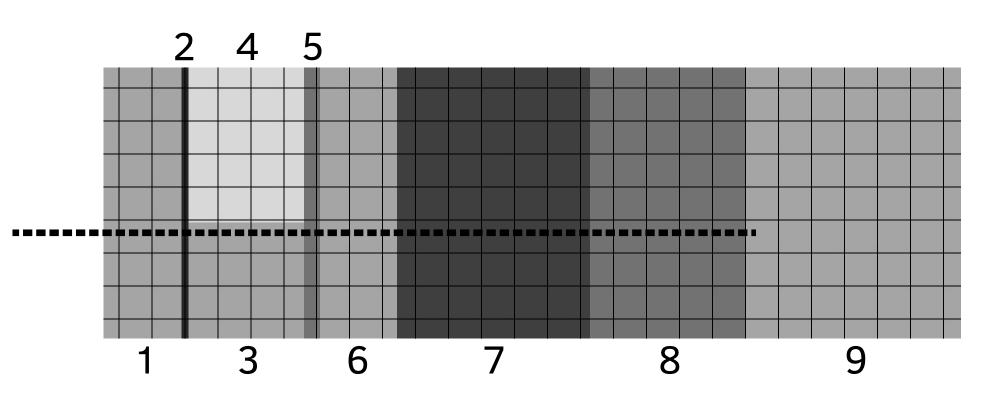Figure 3: Top-down view of the Parodi phantom (Parodi et al.2005), where the shading represents the material densities. Parts 1, 3, 6, 9 are PE; 7 is PMMA; 2 is Bone; 5, 8 are Muscle; 4 is Lung. The beam is illustrated with the dotted line coming in from the left. A voxelized version of this image is created, with a ${2}^{3}$ mm${}^{3}$ voxel size.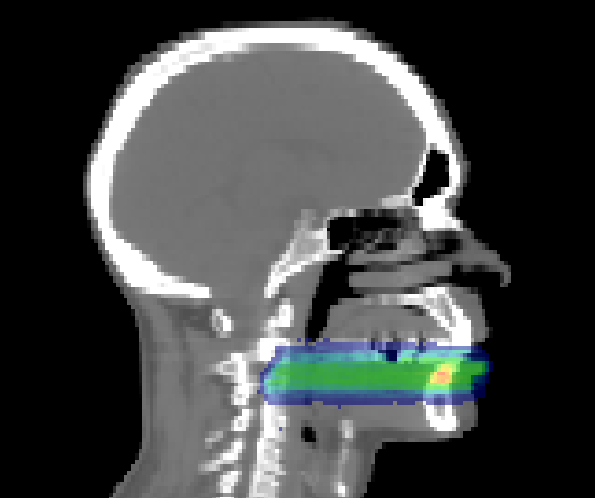Figure 4: Sagittal view of the patient CT image, illustrated with the PG yield caused by the associated treatment plan. A beam from an original treatment plan has been rotated to align with the image axes, to both make projections easier and to increase the heterogeneity of the materials and thereby increase the challenge to vpgTLE. The applied radiation is the distal layer of one of the beams of the original plan so that the distal falloﬀ is better deﬁned in contrast to the spread out Bragg Peak (SOBP). The voxel size is again ${2}^{3}$ mm${}^{3}$.

##### 2.2.2 Bias

To establish the presence of bias, as function of spatial or spectral dimensions, the relative diﬀerence with respect to the reference is investigated. In addition, certain subregions are studied separately for Test case 1, because material-based regions can easily be isolated.

##### 2.2.3 Eﬃciency, Gain and Convergence

An important quantity that characterizes a variance reduction method is the eﬃciency ${𝜖}_{k}$, and is computed by considering the time $t$ required to reach a variance ${\sigma }_{k}^{2}$ per voxel $k$, see eq. 4. Comparing the ratio of eﬃciencies of two methods gives a quantiﬁed gain $G$ (eq. 5, where $TLE$ and $A$ refer to vpgTLE and analog MC respectively). Using a measure of centrality (e.g. mean, median) on the gains per voxel ${G}_{k}$, a global measure for the eﬃcacy of vpgTLE is obtained. A histogram of the gains within an image is presented to have an idea of the worst and best case performance of vpgTLE.

 ${𝜖}_{TLE,k}=\frac{1}{t×{\sigma }_{TLE,k}^{2}}$ (4)
 $G=\frac{{𝜖}_{TLE,k}}{{𝜖}_{A,k}}$ (5)

The proposed vpgTLE is a variance reduction method: it reduces the variance for any given PG simulation with respect to an analog simulation. As a simulation runs, the output converges, which is to say: its variance reduces. A common measure to ensure suﬃcient convergence is to require that the uncertainty $\sigma$ associated to the quantity of interest is not more than 2% of the signal. A variance reduction technique such as vpgTLE translates into reaching the threshold faster, and therefore a gain with respect to analog MC. We compute the gain therefore both by taking the ratio of the runtime of the two methods at the 2% convergence level, as well as by computing the gain (eq. 5). With the same data, we estimate the total runtime required to produce a suﬃciently converged image.

##### 2.2.4 Inﬂuence of voxel size

An essential property of vpgTLE is the fact it records PG production probabilities all along the primary’s path, instead of waiting for the MC engine to produce a PG. This means that vpgTLE requires much fewer propagated primaries to touch all voxels. This eﬀect could be magniﬁed further when smaller voxels are used. Recent developments (Marcatili et al.2014) in super-resolution or multi-scale CT models involve smaller voxels in order to increase simulation accuracy for smaller or thinner organs such as the rectum, bladder, or spine. We demonstrate the increased beneﬁts of vpgTLE on Test case 2.

##### 2.2.5 Hardware, software, parameters

Gate 7.1 (Jan et al.2004Sarrut et al.2014) with Geant 4.10.01 and the QGSP_BIC_HP_EMY physics list, commonly used for PG studies, are used in this analysis.

In Gate, scorers are deﬁned as actors, which can be attached to volumes, all deﬁned through the Gate macro language. The vpgTLE method was implemented as an actor, that keeps a PG spectrum for each voxel, the number and volume of which can also be controlled through the macro language. A helper actor, that outputs the analog MC in a similar manner as the PGyd was implemented to facilitate analysis. The output was validated against the GatePhaseSpaceActor, a thoroughly used and tested part of Gate. We used a gamma production cut of 3 keV in order to remove a high number of photons that cannot exit the phantom or patient geometry.

Timing information is obtained with the aid of the GateSimulationStatisticActor, executed on a Intel Core i7-3740QM CPU @ 2.70GHz, SpeedStep oﬀ, whilst using a single core. In summary, table 1 lists the main parameters used for the simulations.

Table 1: Summary of vpgTLE analysis parameters
 Test case 1 Test case 2 Beam 160 MeV, disc shaped cross- Distal layer (133.08 MeV) section, Gaussian spatial and of clinical treatment plan angular distr. with $\sigma$ of (7 spots) 3.5 mm, 2 mrad respectively Phantom description Parodi et al. (2005) Clinical head and neck CT Phantom voxel size 2mm PGyd voxel size 2 mm 1, 2, 5 mm PGdb primaries/element $1{0}^{9}$ PGdb max. proton energy 200 MeV Number of PG bins 250 Number of proton bins 250 vpgTLE primary-sets $1{0}^{3}-1{0}^{6}$ Analog primary-sets $1{0}^{6}-1{0}^{9}$ Reference Analog primary-set $1{0}^{9}$ Studied projections Spectral and along beam Extra studies Central voxel line yield Inﬂuence of PGyd voxel size Spatial window 90% yield region Spectral window 1-8 MeV Variance computation 10 batches per primary-set Step size 1 mm

### 3 Results

#### 3.1 Test case 1

First we veriﬁed that vpgTLE yields are identical to the results produced with pgTLE, shown in El Kanawati et al. (2015). Then, we compared our method to the analog reference. Figure 5 depicts the yield on the ﬁrst row, as a function of the depth (left column) and the PG energy (right column). The relative diﬀerence of the PG yield is shown, both integrated over the entire coronal plane (second row) or at the voxel line on the beam path (third row). Row one, a plot of the yield, shows a perfect overlap of vpgTLE with respect to the reference. We must look to the relative diﬀerences of the various vpgTLE outputs with respect to the reference in row 2 to observe any diﬀerences. The shaded areas represent 2$\sigma$ error bands.

With $1{0}^{3}$ primary particles, the mean is noisiest, as expected. An overestimate beyond 170 mm is visible, which is about the location of the Bragg Peak. The average relative diﬀerence over the depth is $4.0×1{0}^{-4}$ along the beam, which is a good performance, but due to the relative diﬀerence with the reference exceeding 1% in the distal region and the very wide error bands, we would argue $1{0}^{3}$ primaries is insuﬃcient for a reliable prediction. The distal systematic shift has reduced when using $1{0}^{4}$ or more primaries. Two regions with bias remain: a consistent overestimate of about 0.5% at around 160 mm depth, and then, past the Bragg Peak, an erratic mean with wide uncertainty bands. The latter can be explained by nuclear events. Once a proton collides and is absorbed, it can no longer produce PGs. Towards the end, the precise number of remaining protons grows more uncertain, and just $1{0}^{3}$ primaries are not enough for a good estimate of the variance. Increasing the number of primaries reduces the uncertainty and improves the mean yield, but the eﬀect remains. The average relative diﬀerence over the depth is of the order of $1{0}^{-4}$ for all primary sets.

The spectral column on the right demonstrates vpgTLE is close to the analog reference over the whole spectrum, with a small ﬂuctuation at the high end of the spectrum. The pattern present for all primary sets must be due to the PGdb, and is the only bias we observe. The average relative diﬀerence varies between $-1.3×1{0}^{-4}$ with $1{0}^{3}$ primaries and $6.0×1{0}^{-5}$ for $1{0}^{6}$ primaries. This supports the hypothesis that we have converged to the bias introduced by the uncertainty of the PGdb. The wide error bands for $1{0}^{3}$ are again visible, with the error bands for $1{0}^{4}$ or more primaries staying within 1% of the mean over the entire range.

The bottom row, the line of voxels centered on the beam path, shows a more erratic behavior. One major diﬀerence is the proximal overestimate and distal underestimate with $1{0}^{3}$ primaries. With $1{0}^{5}$ primaries or less, the average relative diﬀerence is on the order of $1{0}^{-3}$ or more, while $1{0}^{6}$ primaries results in $3.6×1{0}^{-4}$. The uncertainty is, naturally larger. The spectral view is stable and the depth view has an increased variance towards the end of the proton range.

Test case 1: Yield and Relative Diﬀerence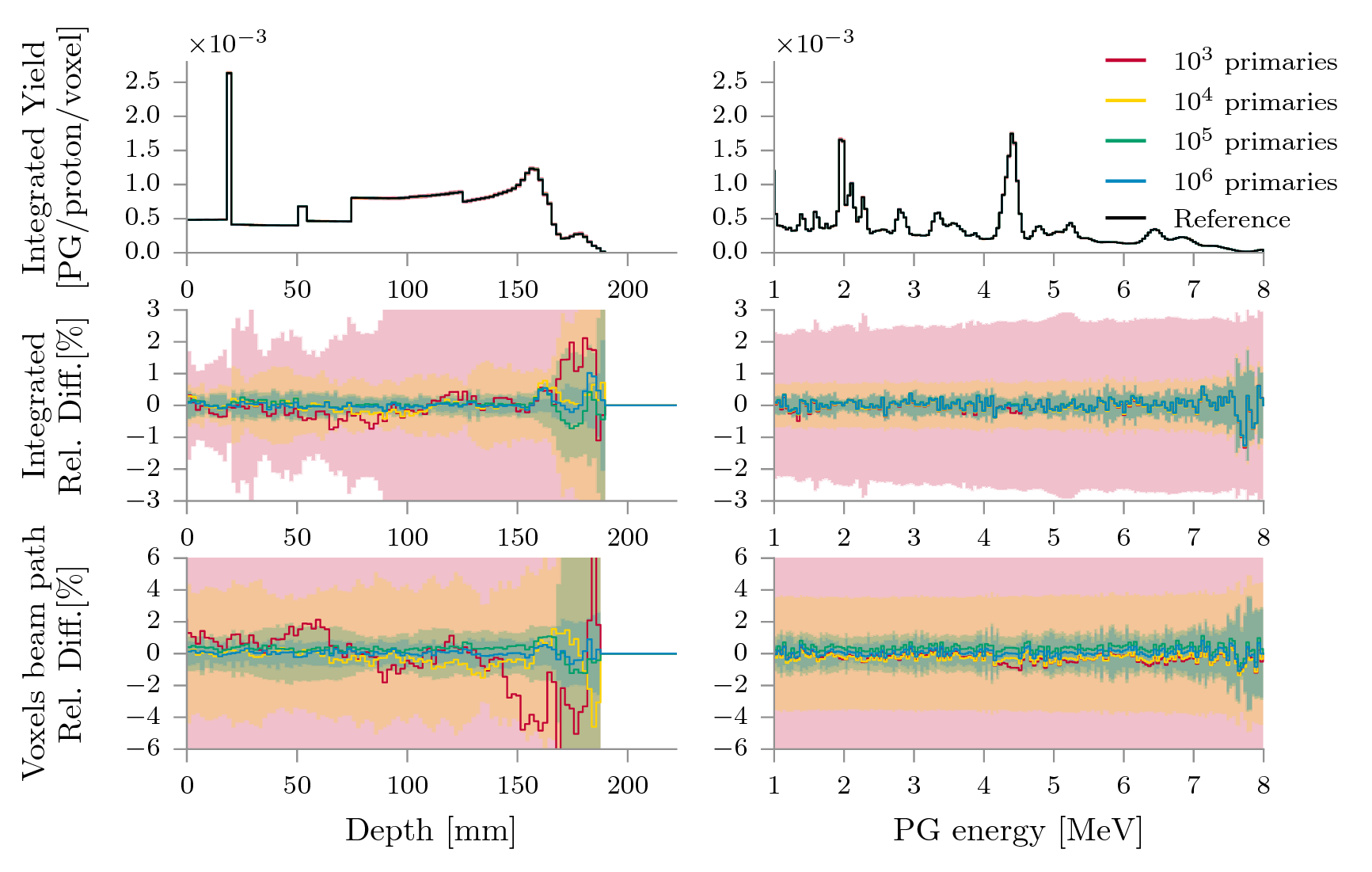Figure 5: Test case 1: Row 1 shows the PG yields and row 2 the relative diﬀerence with respect to the reference ($\frac{vpgTLE-Reference}{Reference}$). The yield corresponds to the mean over 10 simulations. For both rows, note that the yield was integrated over all other dimensions. Row 3 shows the relative diﬀerence on the line of voxels on the center of the beam path, where we did not integrate over all other dimensions. The left column is a projection along the beam-axis, while the right column shows the spectral bins integrated over all voxels considered. The shaded areas represent 2$\sigma$ error bands, where $\sigma$ is the standard deviation over the mean of 10 simulations. Note that the covarying pattern in the relative diﬀerence is due to the noise of the analog signal, and does not represent any issue with the vpgTLE implementation.

Figure 6 shows side-by-side the convergence of the median relative uncertainty and a histogram of the gains computed with eq. 5. We see that a median convergence to within 2% is reached in about 3 minutes and about 68 hours with vpgTLE and analog MC respectively. At the 2% convergence level, the gain is $1.55×1{0}^{3}$. The histograms on the left show that the gains are stable with respect to the number of vpgTLE primaries. That means vpgTLE has no systematic problems. We can clearly see the skew of the distributions (note the logarithmic scale on the $x$-axis). The worst gain is a factor of $6.19×1{0}^{1}$, while the best voxel clocks in at $5.21×1{0}^{4}$, with a median of $1.40×1{0}^{3}$.

Test case 1: Gain distribution and Convergence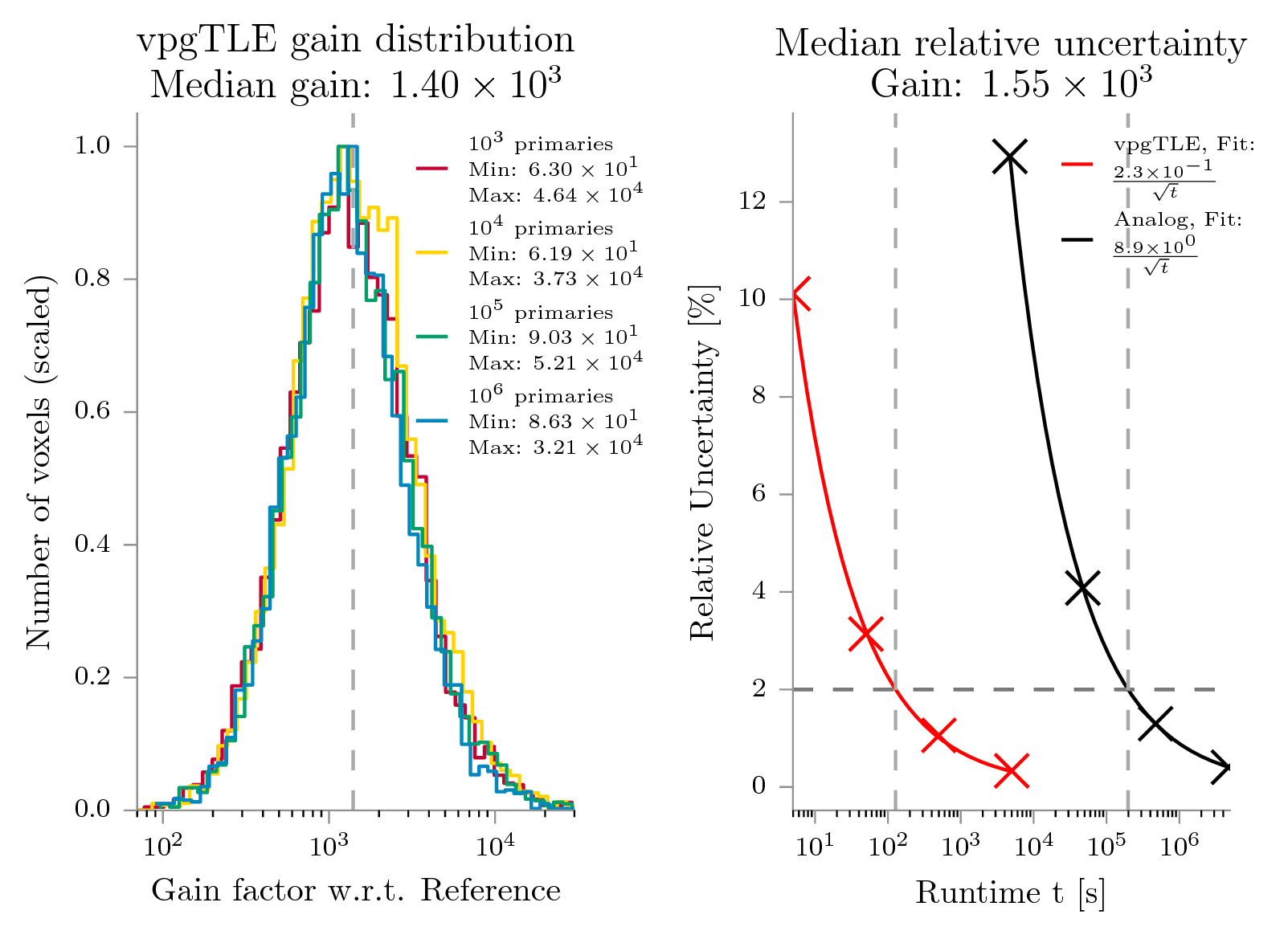Figure 6: Test case 1. Left, the eﬃciency is computed, per voxel, according to eq. 4, for all vpgTLE primary-sets with respect to the reference. Right, the median relative uncertainty as a function of runtime t, for both the analog and vpgTLE methods. Each successive point is generated with $1{0}^{3}$ - $1{0}^{6}$ primaries for vpgTLE, and with $1{0}^{6}$ - $1{0}^{9}$ primaries for analog MC. As t increases, the relative uncertainty decreases as $\frac{C}{\sqrt{t}}$, where $C$ is a ﬁt factor. To compute the gain, we take the ratio of the runtimes at the 2% level, indicated by the dashed horizontal line, generally considered to be suﬃciently converged.

#### 3.2 Test case 2

Look to ﬁgure 7 for the yield and relative diﬀerence of vpgTLE with respect to the reference. The width of the $2\sigma$-bands has increased with respect to Test case 1. Along the beam, left column of the ﬁgure, we see that $1{0}^{3}$ primaries produce an erratic line, while $1{0}^{5}$ and up are close to 0%. Past the distal end, we see signiﬁcant divergence as in Test case 1. While the average bias is of similar magnitude as in Test case 1 ($1{0}^{-4}$, except for $1{0}^{3}$ primaries), on the distal end the bias has not quite dissipated with $1{0}^{6}$ primaries. A likely explanation for the worse performance is the diﬀerent beam with respect to Test case 1: now we look at the yield caused by a full energy layer composed of 7 spots, resulting in the primaries being spread over a larger volume and result therefore in lower statistics per voxel. We can again attribute part of the increase in error to the systematic error induced by the PGdb. The spectral view is as stable as in Test case 1, which is spread over the same 250 bins, and diﬀers only by wider error bands.

Test case 2: Yield and Relative Diﬀerence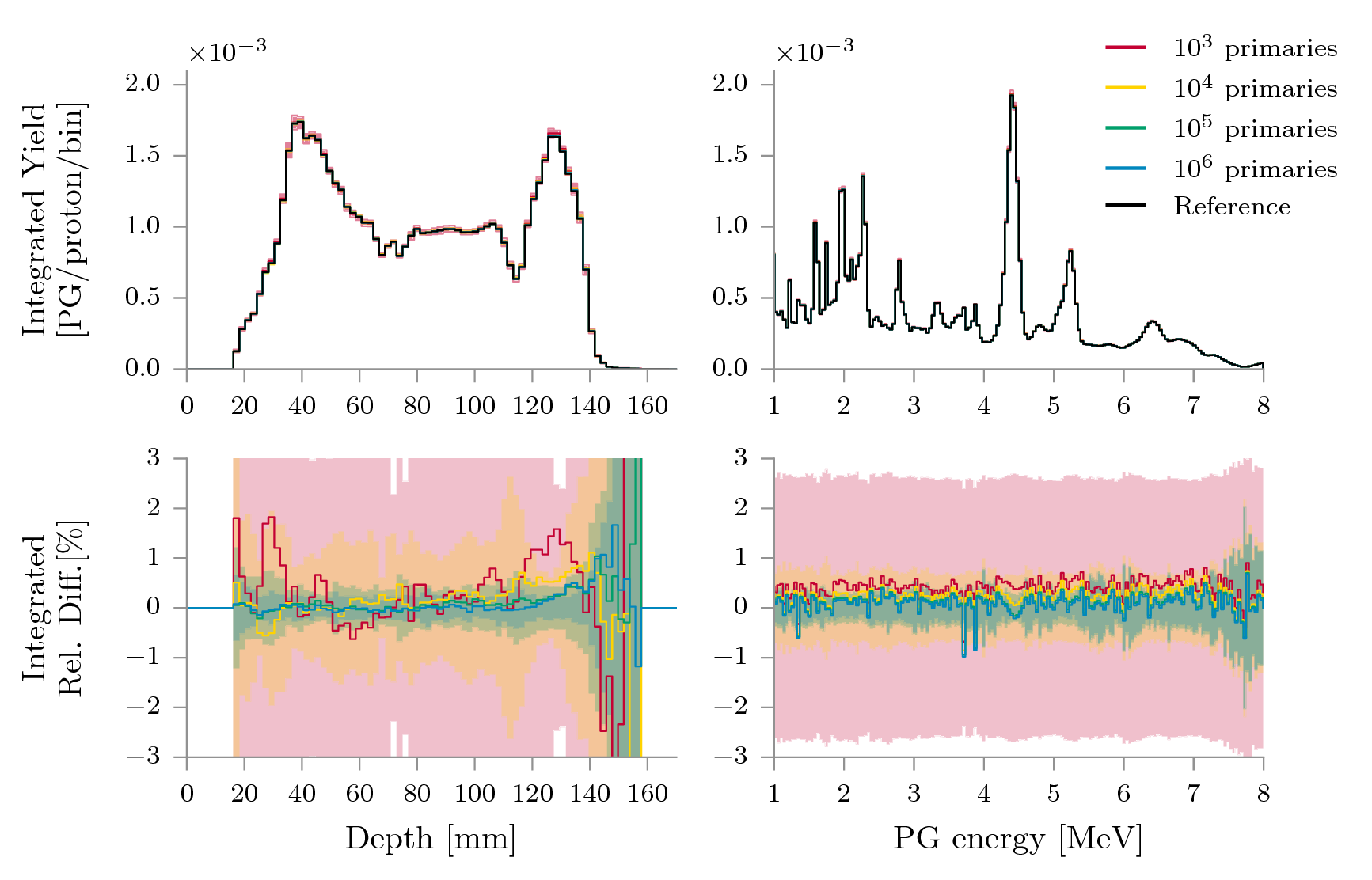Figure 7: Test case 2. Row 1 shows the PG yields and row 2 the relative diﬀerence with respect to the reference ($\frac{vpgTLE-Reference}{Reference}$). For both rows, note that the yield was integrated over all other dimensions. The left column is a projection along the beam-axis, while the right column shows the spectral bins integrated over all voxels considered. The shaded areas represent 2$\sigma$ error bands, where $\sigma$ is the standard deviation over the mean of 10 simulations.

Figure 8 shows the convergence and gain of vpgTLE for Test case 2. The gain is slightly less than in Test case 1. A suﬃciently converged PGyd now requires little over 4 hours on a single core with vpgTLE and about 180 days with analog MC. Excluding the $1{0}^{3}$ primary-set because of its outliers, the worst gain is $2.70×1{0}^{1}$ and the best is $8.96×1{0}^{4}$, with a median gain of $9.98×1{0}^{2}$, and a gain of $1.03×1{0}^{3}$ at 2% relative uncertainty.

Test case 2: Gain distribution and Convergence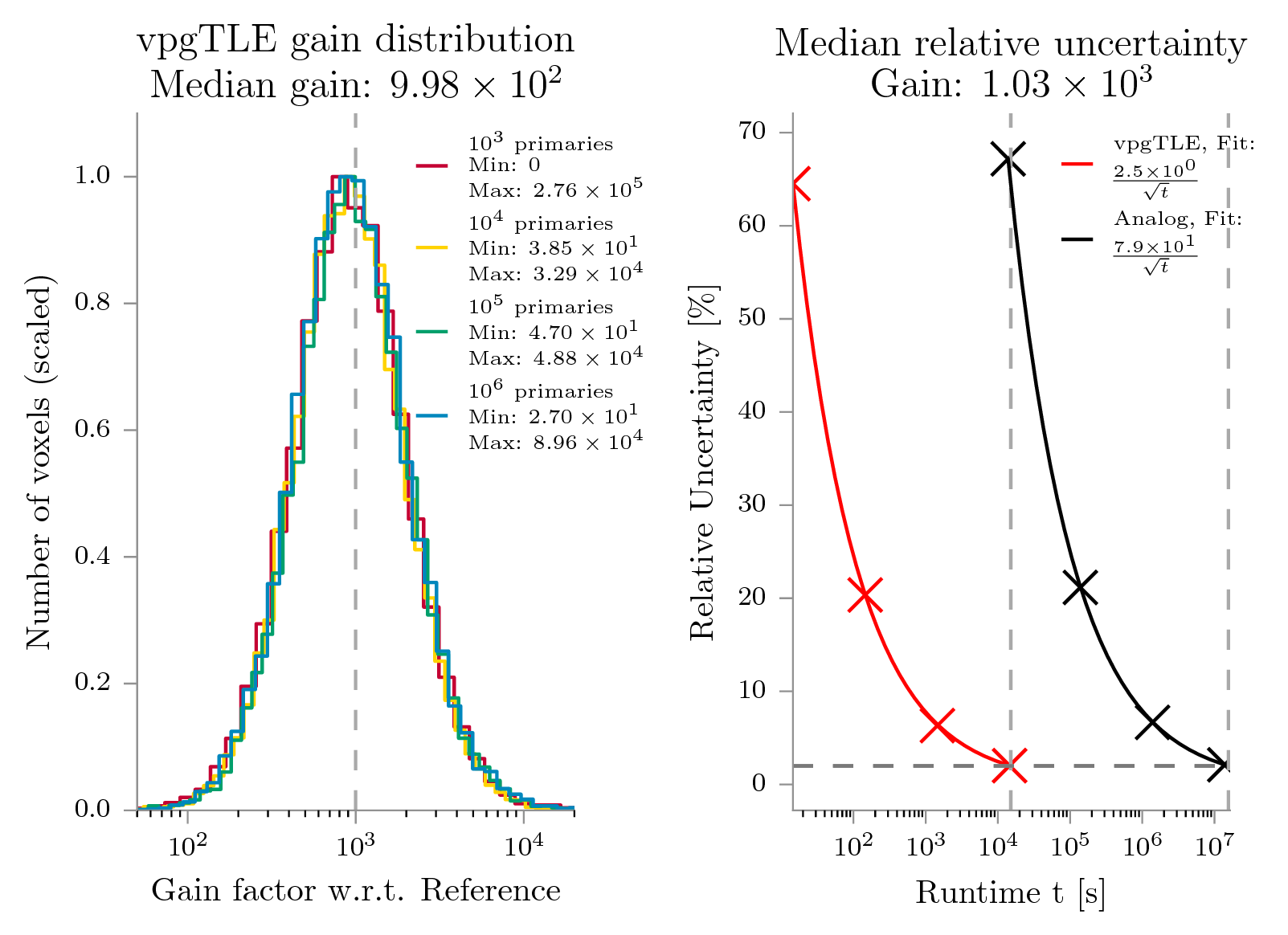Figure 8: Test case 2. Left, the gain histogram is shown, for all vpgTLE primary-sets with respect to the reference. Right, the mean relative uncertainty is plotted as a function of runtime, for both the analog and vpgTLE methods. Each succesive point is generated with $1{0}^{3}$ - $1{0}^{6}$ primaries for vpgTLE, and with $1{0}^{6}$ - $1{0}^{9}$ primaries for analog MC. We take the ratio of the runtimes at the 2% level to obtain the gain.

The last result is the eﬀect of changing the PGyd voxel size on the gain. We have observed in ﬁgures 6 and 8 that the gain distribution is independent of the number of primaries. Hence, in order to save time, we conduct this investigation with $1{0}^{7}$ primaries for analog MC and $1{0}^{4}$ for vpgTLE, which are at a similar level of convergence (see ﬁg. 8). The gain ratio is computed as the ratio between these sets, per voxel size. Due to memory consumption considerations, the minimum voxel size was set at 1 mm${}^{3}$, which results in an image of 1666 MB (833 MB on disk).

Figure 9 shows the gain histograms for each voxel size. It can be seen that the advantage of vpgTLE magniﬁes with respect analog MC as voxel size decreases. This is in agreement with the hypothesis that vpgTLE needs fewer primaries with respect to analog MC to estimate the PG yield. Users interested in super-resolution output can expect higher gains than reported for our two test cases.

Test case 2: gain as function of voxel size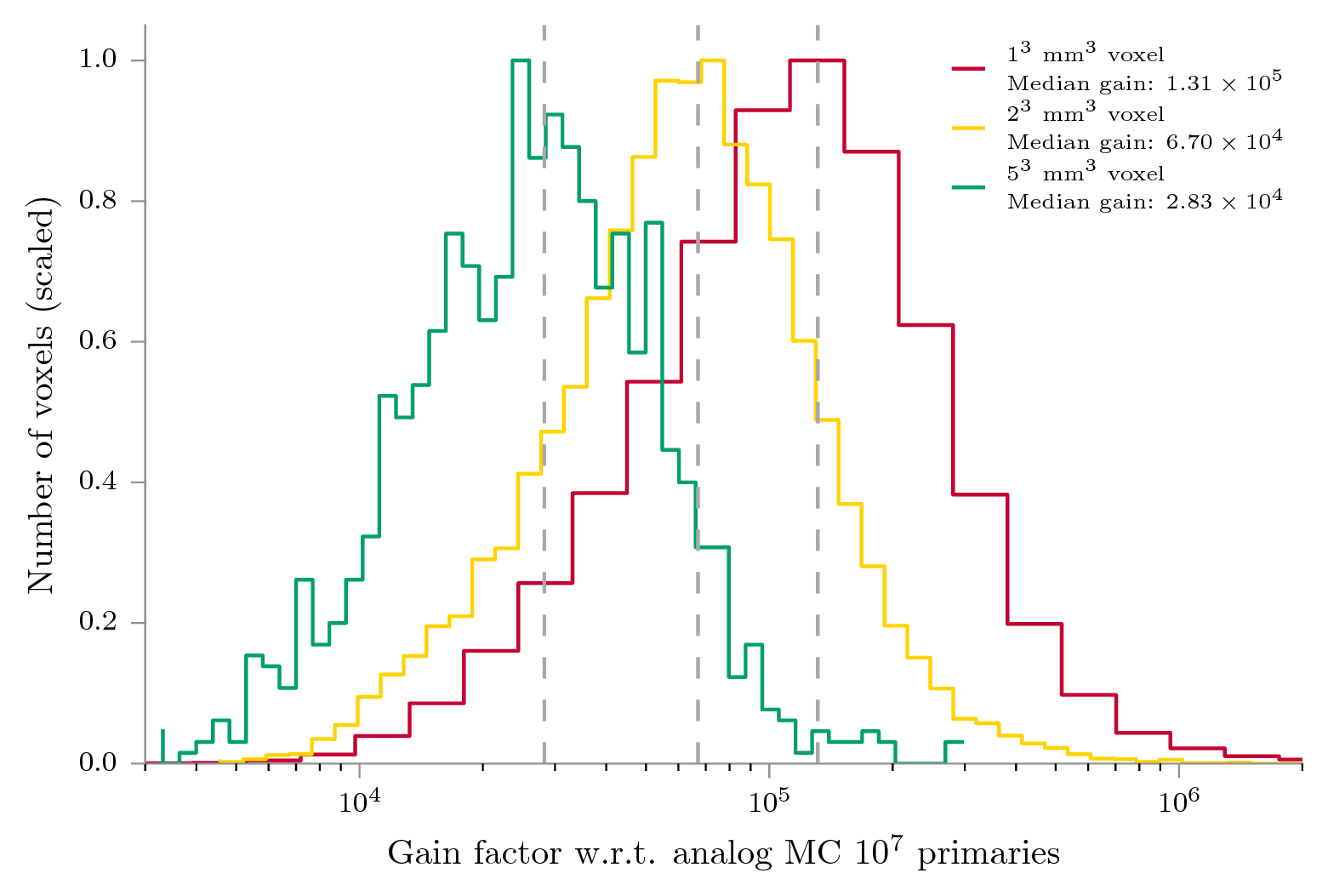Figure 9: The distribution of gains of a vpgTLE simulation ($1{0}^{4}$ primaries) w.r.t. an analog MC ($1{0}^{7}$ primaries) are plotted. The distribution, and the medians, shift up as the voxel size decreases.

### 4 Discussion

The current implementation of vpgTLE stores each bin as a double (64 bit) in memory, and converts to ﬂoat (32 bit) when writing to disk. The memory consumption is therefore linked to the number of PG bins, image and voxel size. By default the vpgTLE actor will copy the size and voxel size of the image it is attached to. As described before, for clinical CT images this will result in on-disk images of tens of GBs, and twice that in memory usage during the simulation. In this paper, we therefore shrank the PGyd volume to a region that envelops the PG production sites with some margin (see ﬁgure 10). This resulted in an on-disk image size of about 104 MB. With 1 mm${}^{3}$ voxels, the image size increases eight-fold to 833 MB (double at runtime: 1666 MB). A PGyd with the size of the CT data used in Test case 2 with 1 mm${}^{3}$ voxels would blow up to 120 GB on disk, 240 GB in memory at runtime.

Storing the intermediate PGyd is similar to the practice of storing intermediate phasespaces in complex accelerator simulations. A nice side-eﬀect of having two stages is that if the user for instance wants to reposition a detector or compare diﬀerent detectors altogether, only the PGs have to be re-propagated.

Test case 2: PGyd output region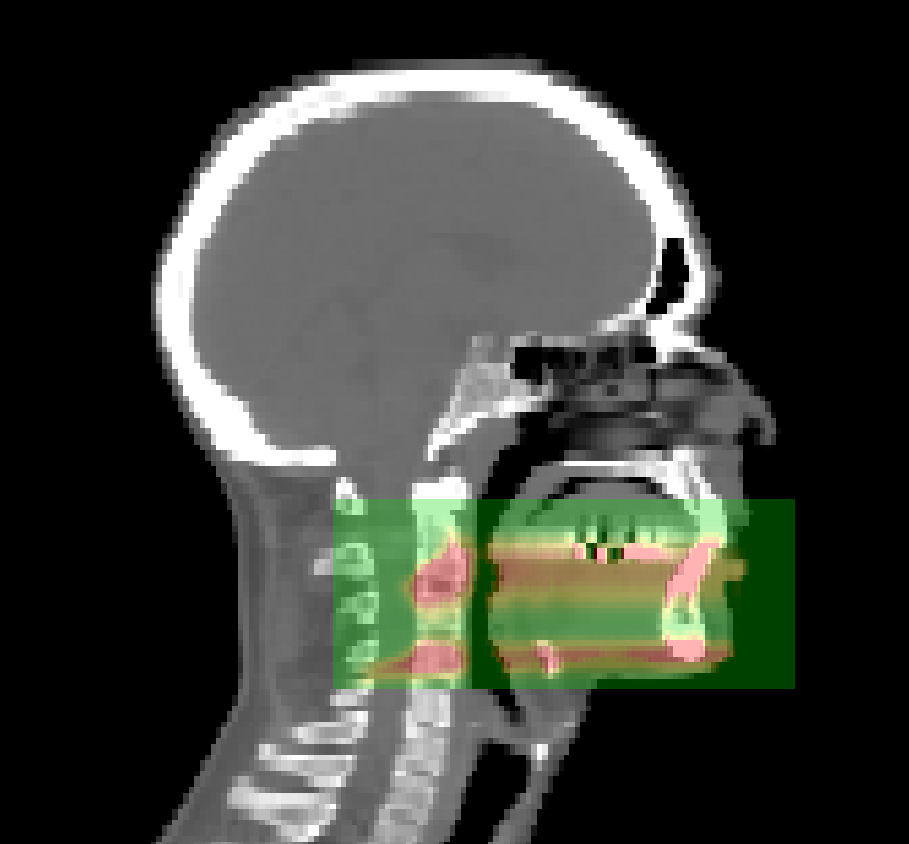Figure 10: The region of the PGyd was set to a smaller region than the patient CT image, in order to reduce memory consumption. The region of the PGyd is visible as green, with the PG production visible as red bands.

Before Stage 1, a number of important parameters are set: the number of primaries per element in the PGdb, the minimum, maximum and number of bins for both the primary and PG energy. Naturally, more primaries increases the quality of the estimate, while more bins spanning a wider range improve the precision, but slow down the convergence to an acceptable mean or median uncertainty. Assuming a maximum primary energy of 250 MeV, we need 250 bins for a precision of 1 MeV. The current implementation has linear binning, so assuming the location of the BP fall-oﬀ is of interest, where the primary energy is lowest, a 1 MeV bin translates to a proton range in water of about 24 $\mu$m, more than enough considering the typical ${2}^{3}$ mm${}^{3}$ voxel size. Note that computing the PGdb for more bins requires more particles to ensure proper bin ﬁlling. It took approximately 1000 days of CPU-time to compute the PGdb used in this study.

#### 4.2 Comparison with other variance reduction techniques

A conventional approach to variance reduction for rare processes is interaction biasing (IB), where the probability of the interaction of interest is multiplied with factor $\alpha$, and is compensated for by decreasing the weight of the continuing track (and secondaries) with factor $\frac{1}{\alpha }$. Parameter $\alpha$ is then chosen such that an interaction occurs once per interval of interest (say, once per voxel). Alternatively, interaction forcing (IF) forces an interaction per interval, and weights any subsequent interaction with the probability that former interaction occurred. As the incident particle may be killed in the process of interest (as is the case for PG production), some implementations (e.g. MCNPX, Geant4, EGSNRC) split the track into a collided and uncollided version to prevent the loss of statistics in the distal part of the track.

Another standard technique for rare processes is particle splitting: instead of producing a single new particle in the interaction of interest, $N$ particles are produced, each with weight $\frac{1}{N}$. This method may be applied in addition to IB or IF. Storing the whole PG spectrum in Stage 1 and sampling it in Stage 2 could be viewed as delayed particle splitting. Not implementing the separation between Stages 1 and 2 would have been possible by directly generating a PG from the spectra computed in Stage 1 as the protons traverse the phantom.

When considering IB, the main disadvantage is the free parameter $\alpha$, which should be chosen in such a way that suﬃciently often a PG is produced. What suﬃciently often means will depend on each individual simulation. Moreover, the PG prediction is still a probabilistic process: in some voxels a PG may be produced, in some may not. IF is much more similar to vpgTLE, in that it gives a deterministic prediction per interval. Having a larger number of calls to the physics processes is the main source of overhead for both IB and IF. For example, in an analog Geant4 simulation, we measure that 70% of computing time is spent in nuclear interactions. In a naive implementation of IB or IF, we run through the nuclear interactions an increased number of times, which indicates that the upper limit of the gain is $\frac{1}{70%}\approx 40%$. Both IB and IF may beneﬁt from precomputed lookup tables to reduce the time spent performing the additional interactions. Lookup tables are most practical if a single or limited number of outputs are sought, e.g. dose, PG.

Indeed, vpgTLE may be considered as a special optimized case of IF with the following diﬀerences:

• the Monte Carlo particle weights are not modiﬁed
• whole inelastic processes are precomputed instead of being called at each interaction
• the complete precomputed gamma energy spectrum is stored

The two last points can also be applied to IF implementations. Hence, PG yields per proton track remains essentially the same between IF and vpgTLE. The diﬀerence may essentially be viewed as conceptual, vpgTLE being inspired by the TLE approach originally put forward by Williamson (1987).

Test case 2: PG spectra as function of depth.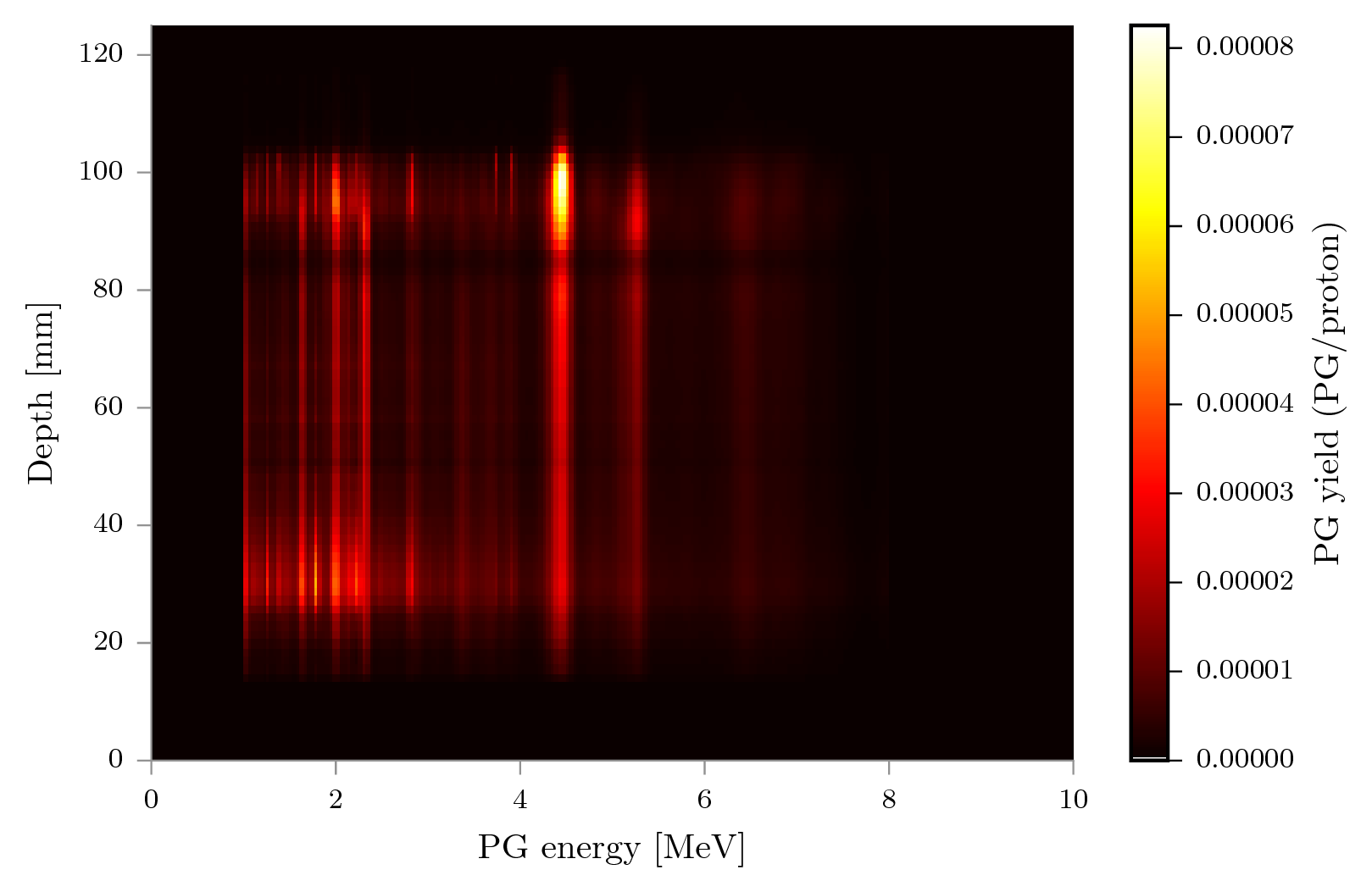Figure 11: vpgTLE allows one to make full use of spatial and spectral information of PGs. As an illustration, this ﬁgure shows a heatmap of PG yields in Test case 2 as function of depth and PG energy, integrated over the transverse dimensions. The ﬁgure might incline a detector developer to tune a spectral camera to the 4.4 MeV peak.

As far as the authors of this paper could establish, the only other published variance reduction technique for PGs is described in Sterpin et al. (2015). This method is fully analytic, incorporating experimental or pretabulated PG emission data and a model that assumes the PG emission region can be modeled similar to the dose in pencil beams. The method raytraces the materials touched by a pencil beam spot, and computes the expected 1D PG proﬁle by a weighted sum of pregenerated proﬁles per material, which takes 0.3 to 10 seconds. The authors admit that this approach does not deal very well with lateral inhomogeneity, a problem that vpgTLE does not have due to TLE methods in principle being assumption free. Another beneﬁt of vpgTLE is the shape of its output: a 4D image where for each voxel a PG spectrum is recorded. This permits the incorporation of the method in many diﬀerent kinds of PG detector simulations, not just detectors that measure the range. As a small example, vpgTLE can be used to investigate the origin of the global spectrum as function of depth along the beam path in ﬁgure 11. vpgTLE will work with any collimated or Compton camera design, PG spectroscopy, or any future detector that takes advantage of spatial or spectral components. Moreover, vpgTLE does not assume anything about the proton ﬂux: it uses Stage 1 to estimate it. The diﬃculty estimating PG yields due to beam spots that produce diﬀerent ranges due to lateral inhomogeneity is therefore avoided: vpgTLE is as sensitive to inhomogeneity as regular MC.

#### 4.3 Background estimation

vpgTLE does not estimate other physical quantities than PGs produced in the target, which means no estimate of the background noise can be obtained with this method. For any detector seeking actual application, no analysis is complete without considering the signal-to-background ratio, and methods to improve it. The background consists of tertiary gammas, mainly produced by secondary neutrons undergoing nuclear interactions, in the target but also in elements of the beamline, other objects in the room such as the patient table, and the detector itself (Pinto et al.2014). Obtaining the background from simulation is problematic for two reasons:

1.
No MC tool correctly estimates the background (see Pinto et al. (2014) section 3.1.2, Sterpin et al. (2015) section IV.A.4). Nuclear reaction models are continuously improved, but as far as we know not speciﬁcally for PG background at this time.
2.
The computing time required for full room simulations is prohibitive, which is why room modeling is left out. Even without room components, the simulation runtime is still long using analog MC, as there are no variance reduction techniques for PG background.

Depending on the purpose of the simulation, various ways of dealing with the lack of reliable MC background prediction are considered. Sterpin et al. (2015), concerned with treatment prediction, deals with the background by adding an oﬀset parameter to their ﬁtting procedure of the PG proﬁles and accept the absence of background estimation in their fast PG method. A procedure like this could be applied for other devices. Simulations for camera optimization, eﬀectively signal-to-background optimization for a multitude of conﬁgurations, also require a background estimate. Because the background may depend on camera parameters and obtaining a measured background for each conﬁguration is not tractable, most groups limit the number of conﬁgurations. The multi-slit camera optimization of Pinto et al. (2014), which correctly does consider hundreds of conﬁgurations, was performed with a manual correction of a single background measurement.

Hence, the fact that in current practice the background is measured or modeled separately, puts vpgTLE at no particular disadvantage compared to other variance reduction methods or analog MC. However, if physics models could give an accurate estimate of the background, a generalized vpgTLE, most urgently of neutrons and neutron induced PGs, might be a possible avenue for variance reduction of the background estimate. The scorer could be attached to not only the target but other objects in the treatment room.

#### 4.4 Future improvements

We did not take into account the systematic error (caused by the PGdb) in our analysis. The PGdb is computed by shooting protons of an energy at least as high as used in the treatment plan into a box of a certain material. This means that at low energies, the PGdb statistics are not as good as at higher energies, meaning that we have a systematic error around the BP region (where the proton energy is lowest). We might therefore consider to supplement our PGdb with the output of a second, low energy proton beam, simply to reduce the systematic error in the BP range. Since we compute the PGdb once, this has no eﬀect on the eﬃciency of the vpgTLE method. It might also be possible to ﬁll the database by querying Geant4 for the cross section at the respective bins. We did implement outputs for the analytic systematic and random error output as laid out in El Kanawati et al. (2015), but Stage 2 does not propagate these errors yet, which would be required for a quantitative analysis of the outputs of Stage 2. Moreover, we found that this analytic error estimate underestimates with respect to the batch method, because it assumes the independence of the track lengths and proton energies, a type of problem IF or IB techniques would not have. Therefore, at this time, the variance of vpgTLE can only be obtained by employ of the batch method.

A thorough analysis of the sparseness of the PGyd has not been conducted, but there is a likely opportunity for memory optimization here. Another option is to reduce the dimensionality of the image to that which the user requires. Users investigating their collimated camera may for example set the PG spectrum to a narrower window and a coarser binning, reducing memory consumption accordingly.

Precomputing an eﬀective linear production coeﬃcient, the main principle of vpgTLE, could be performed for other particles. Adding for instance an eﬀective linear neutron production coeﬃcient may supplement the vpgTLE output with a correct estimate of neutron-induced gamma noise in a PG detector, giving an indication of the background, not just the PG signal. However, such an addition must be investigated to obtain the real eﬃciency.

### 5 Conclusion

vpgTLE is a generic drop-in alternative for computing the expected PG output in voxelized geometries. The method has a ﬁxed memory requirement (a 4D image) with a typical memory size of the order of a few hundred MB. The method reaches a global gain factor of $1{0}^{3}$ for a clinical CT image and treatment plan with respect to analog MC. A median convergence of 2% for the most distal energy layer is reached in approximately four hours on a single core, at which point the output has stabilized to within $1{0}^{-4}$ of an analog reference simulation, when the range or the spectrum is considered. The authors think the method is of interest to those developing and simulating PG detection devices, as well as clinicians studying complex clinical cases that require the precision and accuracy of MC level simulations not oﬀered by analytic algorithms.

The vpgTLE method is open source and fully integrated in Gate. It is available from release 7.2 onwards.

### 6 Acknowledgements

This work was partly supported by Labex PRIMES ANR-11-LABX-0063, t-Gate ANR-14-CE23-0008, France Hadron ANR-11-INBS-0007 and LYric INCa-DGOS-4664. We thank Denis Dauvergne for his assistance with preparing the copy for this paper and Marc Verderi of the Geant4 collaboration for the discussions on variance reduction in Geant4.

### References

Aleksandra K Biegun, Enrica Seravalli, Patrícia Cambraia Lopes, Ilaria Rinaldi, Marco Pinto, David C Oxley, Peter Dendooven, Frank Verhaegen, Katia Parodi, Paulo Crespo, and Dennis R Schaart. Time-of-ﬂight neutron rejection to improve prompt gamma imaging for proton range veriﬁcation: a simulation study. Physics in medicine and biology, 57 (20):6429–44, oct 2012. ISSN 1361-6560. doi: 10.1088/0031-_9155/57/20/6429. URL http://www.ncbi.nlm.nih.gov/pubmed/22996154.

W El Kanawati, J M Létang, D Dauvergne, M Pinto, D Sarrut, É Testa, and N Freud. Monte Carlo simulation of prompt $\gamma$-ray emission in proton therapy using a speciﬁc track length estimator. Physics in Medicine and Biology, 60(20):8067–8086, 2015. ISSN 0031-9155. doi: 10.1088/0031-_9155/60/20/8067. URL http://stacks.iop.org/0031-_9155/60/i=20/a=8067?key=crossref.d38c03af2b13b06ae763a331d1d25dd2.

Christian Golnik, Fernando Hueso-González, Andreas Müller, Peter Dendooven, Wolfgang Enghardt, Fine Fiedler, Thomas Kormoll, Katja Roemer, Johannes Petzoldt, Andreas Wagner, and Guntram Pausch. Range assessment in particle therapy based on prompt $\gamma$-ray timing measurements. Physics in Medicine and Biology, 59(18):5399–5422, sep 2014. ISSN 0031-9155. doi: 10.1088/0031-_9155/59/18/5399. URL http://stacks.iop.org/0031-_9155/59/i=18/a=5399?key=crossref.5437fcd3059992135ec2113679c7dad6.

P Gueth, D Dauvergne, N Freud, J M Létang, C Ray, E Testa, and D Sarrut. Machine learning-based patient speciﬁc prompt-gamma dose monitoring in proton therapy. Physics in medicine and biology, 58(13):4563–77, jul 2013. ISSN 1361-6560. doi: 10.1088/0031-_9155/58/13/4563. URL http://www.ncbi.nlm.nih.gov/pubmed/23771015.

L. G. Henyey and J. L. Greenstein. Diﬀuse radiation in the Galaxy. Astrophysical Journal, 93: 70–83, 1941. doi: 10.1086/144246.

S Jan, G Santin, D Strul, S Staelens, K Assié, D Autret, S Avner, R Barbier, M Bardiès, P M Bloomﬁeld, D Brasse, V Breton, P Bruyndonckx, I Buvat, A F Chatziioannou, Y Choi, Y H Chung, C Comtat, D Donnarieix, L Ferrer, S J Glick, C J Groiselle, D Guez, P-F Honore, S Kerhoas-Cavata, A S Kirov, V Kohli, M Koole, M Krieguer, D J van der Laan, F Lamare, G Largeron, C Lartizien, D Lazaro, M C Maas, L Maigne, F Mayet, F Melot, C Merheb, E Pennacchio, J Perez, U Pietrzyk, F R Rannou, M Rey, D R Schaart, C R Schmidtlein, L Simon, T Y Song, J-M Vieira, D Visvikis, R Van de Walle, E Wieërs, and C Morel. GATE: a simulation toolkit for PET and SPECT. Physics in Medicine and Biology, 49(19):4543, 2004. URL http://stacks.iop.org/0031-_9155/49/i=19/a=007.

F M F C Janssen, G Landry, P Cambraia Lopes, G Dedes, J Smeets, D R Schaart, K Parodi, and F Verhaegen. Factors inﬂuencing the accuracy of beam range estimation in proton therapy using prompt gamma emission. Physics in medicine and biology, 59(15):4427–41, aug 2014. ISSN 1361-6560. doi: 10.1088/0031-_9155/59/15/4427. URL http://www.ncbi.nlm.nih.gov/pubmed/25049223.

Antje-Christin Knopf and Antony Lomax. In vivo proton range veriﬁcation: a review. Physics in medicine and biology, 58(15):R131–60, aug 2013. ISSN 1361-6560. doi: 10.1088/0031-_9155/58/15/ R131. URL http://www.ncbi.nlm.nih.gov/pubmed/23863203.

Shunsuke Kurosawa, Hidetoshi Kubo, Kazuki Ueno, Shigeto Kabuki, Satoru Iwaki, Michiaki Takahashi, Kojiro Taniue, Naoki Higashi, Kentaro Miuchi, Toru Tanimori, Dogyun Kim, and Jongwon Kim. Prompt gamma detection for range veriﬁcation in proton therapy. Current Applied Physics, 12(2):364–368, 2012. ISSN 15671739. doi: 10.1016/j.cap.2011.07.027. URL http://dx.doi.org/10.1016/j.cap.2011.07.027.

Patricia Cambraia Lopes, Enrico Clementel, Paulo Crespo, Sebastien Henrotin, Jan Huizenga, Guillaume Janssens, Katia Parodi, Damien Prieels, Frauke Roellinghoﬀ, Julien Smeets, Frederic Stichelbaut, and Dennis R Schaart. Time-resolved imaging of prompt-gamma rays for proton range veriﬁcation using a knife-edge slit camera based on digital photon counters. Physics in Medicine and Biology, 60(15):6063, 2015. ISSN 0031-9155. doi: 10.1088/0031-_9155/60/15/6063. URL http://iopscience.iop.org/0031-_9155/60/15/6063/article/.

S Marcatili, D Villoing, M P Garcia, and M Bardiès. Multi-scale hybrid models for radiopharmaceutical dosimetry with Geant4. Physics in Medicine and Biology, 59(24):7625–7641, 2014. ISSN 1361-6560. doi: 10.1088/0031-_9155/59/24/7625. URL http://iopscience.iop.org/0031-_9155/59/24/7625/article/.

Chul Hee Min, Chan Hyeong Kim, Min Young Youn, and Jong Won Kim. Prompt gamma measurements for locating the dose falloﬀ region in the proton therapy. Applied Physics Letters, 89 (18):38–41, 2006. ISSN 00036951. doi: 10.1063/1.2378561.

M Moteabbed, S España, and H Paganetti. Monte Carlo patient study on the comparison of prompt gamma and PET imaging for range veriﬁcation in proton therapy. Physics in medicine and biology, 56(4):1063–82, feb 2011. ISSN 1361-6560. doi: 10.1088/0031-_9155/56/4/012. URL http://www.ncbi.nlm.nih.gov/pubmed/21263174.

Katia Parodi, Falk Pönisch, and Wolfgang Enghardt. Experimental study on the feasibility of in-beam PET for accurate monitoring of proton therapy. IEEE Transactions on Nuclear Science, 52 (C):778–786, 2005. ISSN 00189499. doi: 10.1109/TNS.2005.850950.

I Perali, a Celani, L Bombelli, C Fiorini, F Camera, E Clementel, S Henrotin, G Janssens, D Prieels, F Roellinghoﬀ, J Smeets, F Stichelbaut, and F Vander Stappen. Prompt gamma imaging of proton pencil beams at clinical dose rate. Physics in Medicine and Biology, 59 (19):5849–5871, oct 2014. ISSN 0031-9155. doi: 10.1088/0031-_9155/59/19/5849. URL http://stacks.iop.org/0031-_9155/59/i=19/a=5849?key=crossref.d1c598721e0b970b36f1c8a6ad1dd1a1.

M Pinto, D Dauvergne, N Freud, J Krimmer, J M Letang, C Ray, F Roellinghoﬀ, and E Testa. Design optimisation of a TOF-based collimated camera prototype for online hadrontherapy monitoring. Physics in medicine and biology, 59(24):7653–7674, 2014. ISSN 1361-6560. doi: 10.1088/0031-_9155/ 59/24/7653. URL http://www.ncbi.nlm.nih.gov/pubmed/25415207.

M Pinto, M Bajard, S Brons, M Chevallier, D Dauvergne, G Dedes, M De Rydt, N Freud, J Krimmer, C La Tessa, J M Létang, K Parodi, R Pleskač, D Prieels, C Ray, I Rinaldi, F Roellinghoﬀ, D Schardt, E Testa, and M Testa. Absolute prompt-gamma yield measurements for ion beam therapy monitoring. Physics in Medicine and Biology, 60(2):565–594, 2015. ISSN 0031-9155. doi: 10.1088/0031-_9155/60/2/565. URL http://stacks.iop.org/0031-_9155/60/i=2/a=565?key=crossref.c4760640602341dfb0ce09b91884d4e4.

Marco Pinto, Denis Dauvergne, Nicolas Freud, Jochen Krimmer, Jean M. Létang, and Etienne Testa. Assessment of Geant4 Prompt-Gamma Emission Yields in the Context of Proton Therapy Monitoring. Frontiers in Oncology, 6(January):1–7, 2016. ISSN 2234-943X. doi: 10.3389/fonc.2016. 00010. URL http://journal.frontiersin.org/Article/10.3389/fonc.2016.00010/abstract.

Christian Richter, Guntram Pausch, Steﬀen Barczyk, Marlen Priegnitz, Isabell Keitz, Julia Thiele, Julien Smeets, Francois Vander Stappen, Luca Bombelli, Carlo Fiorini, Lucian Hotoiu, Irene Perali, Damien Prieels, Wolfgang Enghardt, and Michael Baumann. First clinical application of a prompt gamma based in vivo proton range veriﬁcation system. Radiotherapy and Oncology, 118(2):232–237, 2016. ISSN 18790887. doi: 10.1016/j.radonc.2016.01.004. URL http://linkinghub.elsevier.com/retrieve/pii/S0167814016000074.

C Robert, G Dedes, G Battistoni, T T Böhlen, I Buvat, F Cerutti, M P W Chin, a Ferrari, P Gueth, C Kurz, L Lestand, a Mairani, G Montarou, R Nicolini, P G Ortega, K Parodi, Y Prezado, P R Sala, D Sarrut, and E Testa. Distributions of secondary particles in proton and carbon-ion therapy: a comparison between GATE/Geant4 and FLUKA Monte Carlo codes. Physics in medicine and biology, 58(9):2879–99, may 2013. ISSN 1361-6560. doi: 10.1088/0031-_9155/58/9/2879. URL http://www.ncbi.nlm.nih.gov/pubmed/23571094.

F. Roellinghoﬀ, M. H. Richard, M. Chevallier, J. Constanzo, D. Dauvergne, N. Freud, P. Henriquet, F. Le Foulher, J. M. Létang, G. Montarou, C. Ray, E. Testa, M. Testa, and a. H. Walenta. Design of a Compton camera for 3D prompt-$\gamma$ imaging during ion beam therapy. Nuclear Instruments and Methods in Physics Research, Section A: Accelerators, Spectrometers, Detectors and Associated Equipment, 648 (SUPPL. 1):S20–S23, aug 2011. ISSN 01689002. doi: 10.1016/j.nima.2011.01.069. URL http://linkinghub.elsevier.com/retrieve/pii/S0168900211001471.

F Roellinghoﬀ, a Benilov, D Dauvergne, G Dedes, N Freud, G Janssens, J Krimmer, J M Létang, M Pinto, D Prieels, C Ray, J Smeets, F Stichelbaut, and E Testa. Real-time proton beam range monitoring by means of prompt-gamma detection with a collimated camera. Physics in medicine and biology, 59(5):1327–38, 2014. ISSN 1361-6560. doi: 10.1088/0031-_9155/59/5/1327. URL http://www.ncbi.nlm.nih.gov/pubmed/24556873.

David Sarrut, Manuel Bardiès, Nicolas Boussion, Nicolas Freud, Sébastien Jan, Jean-Michel Létang, George Loudos, Lydia Maigne, Sara Marcatili, Thibault Mauxion, Panagiotis Papadimitroulas, Yann Perrot, Uwe Pietrzyk, Charlotte Robert, Dennis R Schaart, Dimitris Visvikis, and Irène Buvat. A review of the use and potential of the GATE Monte Carlo simulation code for radiation therapy and dosimetry applications. Medical Physics, 41(6), 2014. doi: http://dx.doi.org/10.1118/1.4871617. URL http://scitation.aip.org/content/aapm/journal/medphys/41/6/10.1118/1.4871617.

Eric Sheldon and Douglas M Van Patter. Compound Inelastic Nucleon and Gamma-Ray Angular Distributions for Even- and Odd-Mass Nuclei. Rev. Mod. Phys., 38(1):143–186, jan 1966. doi: 10.1103/RevModPhys.38.143. URL http://link.aps.org/doi/10.1103/RevModPhys.38.143.

J Smeets, F Roellinghoﬀ, D Prieels, F Stichelbaut, A Benilov, P Busca, C Fiorini, R Peloso, M Basilavecchia, T Frizzi, J C Dehaes, and A Dubus. Prompt gamma imaging with a slit camera for real-time range control in proton therapy. Physics in medicine and biology, 57(11):3371–405, 2012. ISSN 1361-6560. doi: 10.1088/0031-_9155/57/11/3371. URL http://www.ncbi.nlm.nih.gov/pubmed/22572603.

E Sterpin, G Janssens, J Smeets, François Vander Stappen, D Prieels, Marlen Priegnitz, Irene Perali, and S Vynckier. Analytical computation of prompt gamma ray emission and detection for proton range veriﬁcation. Physics in Medicine and Biology, 60 (12):4915–4946, 2015. ISSN 0031-9155. doi: 10.1088/0031-_9155/60/12/4915. URL http://stacks.iop.org/0031-_9155/60/i=12/a=4915?key=crossref.aabd8815e135401a22d165e343a7bac4.

E. Testa, M. Bajard, M. Chevallier, D. Dauvergne, F. Le Foulher, N. Freud, J. M. ??tang, J. C. Poizat, C. Ray, and M. Testa. Monitoring the Bragg peak location of 73 MeVu carbon ions by means of prompt ?? -ray measurements. Applied Physics Letters, 93(9):1–10, 2008. ISSN 00036951. doi: 10. 1063/1.2975841. URL http://arxiv.org/abs/0809.0185http://dx.doi.org/10.1063/1.2975841.

Mauro Testa, Joost M Verburg, Mark Rose, Chul Hee Min, Shikui Tang, El Hassane Bentefour, Harald Paganetti, and Hsiao-Ming Lu. Proton radiography and proton computed tomography based on time-resolved dose measurements. Physics in Medicine and Biology, 58(22):8215–8233, nov 2013. ISSN 0031-9155. doi: 10.1088/0031-_9155/58/22/8215. URL http://stacks.iop.org/0031-_9155/58/i=22/a=8215?key=crossref.9766466f3cf307946adae72478a2726d.

Joost M Verburg and Joao Seco. Proton range veriﬁcation through prompt gamma-ray spectroscopy. Physics in Medicine and Biology, 59(23): 7089–7106, 2014. ISSN 0031-9155. doi: 10.1088/0031-_9155/59/23/7089. URL http://stacks.iop.org/0031-_9155/59/i=23/a=7089?key=crossref.2c380dcfa8360cb6e6defe62dd49f24a.

Joost M Verburg, Helen a Shih, and Joao Seco. Simulation of prompt gamma-ray emission during proton radiotherapy. Physics in Medicine and Biology, 57(17):5459–5472, sep 2012. ISSN 0031-9155. doi: 10.1088/0031-_9155/57/17/5459. URL http://www.ncbi.nlm.nih.gov/pubmed/22864267.

Joost M Verburg, Kent Riley, Thomas Bortfeld, and Joao Seco. Energy- and time-resolved detection of prompt gamma-rays for proton range veriﬁcation. Physics in medicine and biology, 58(20):L37–49, oct 2013. ISSN 1361-6560. doi: 10.1088/0031-_9155/58/20/L37. URL http://www.ncbi.nlm.nih.gov/pubmed/24077338.

J F Williamson. Monte Carlo evaluation of kerma at a point for photon transport problems. Medical physics, 14(4):567–576, 1987. ISSN 00942405. doi: 10.1118/1.596069.